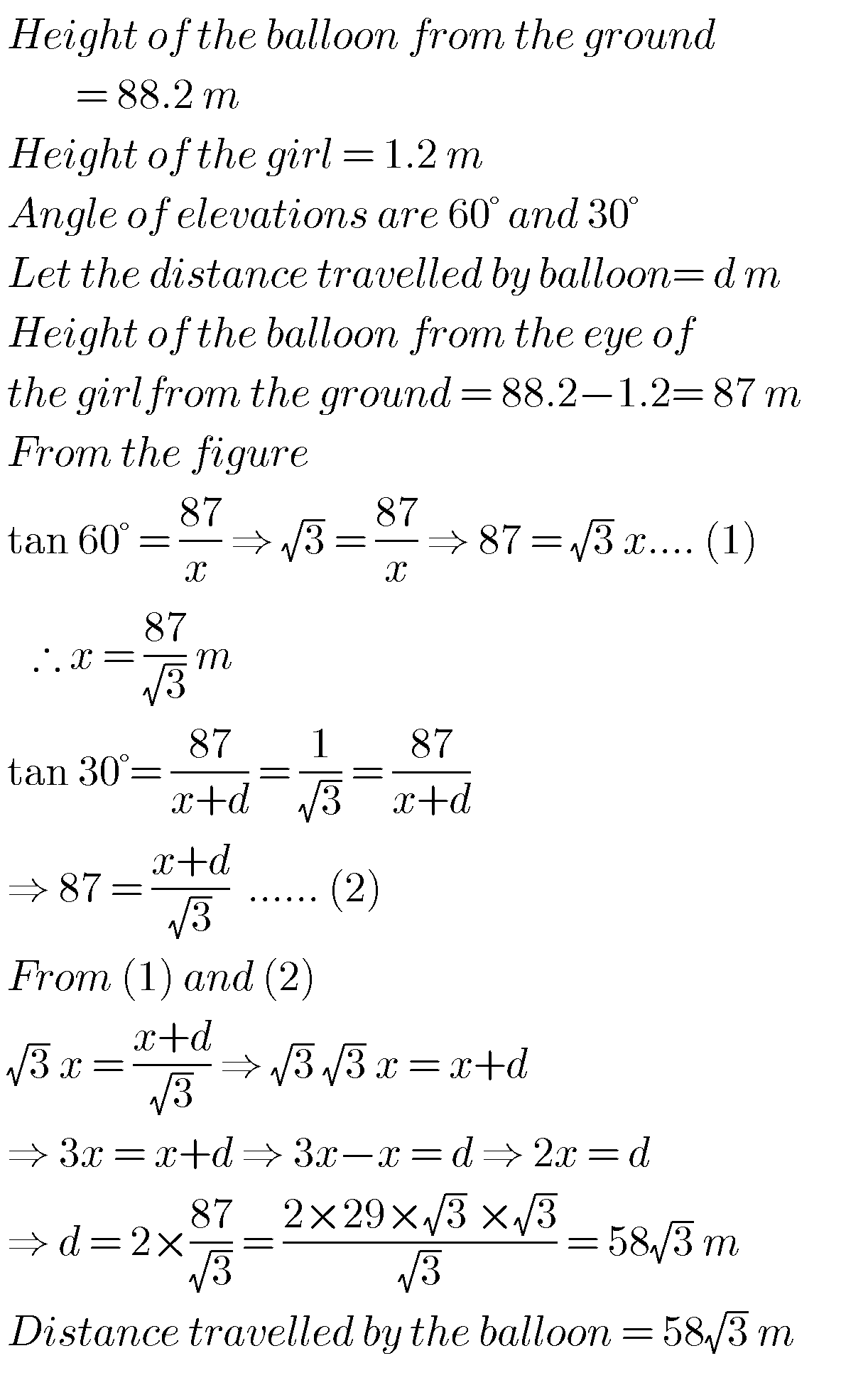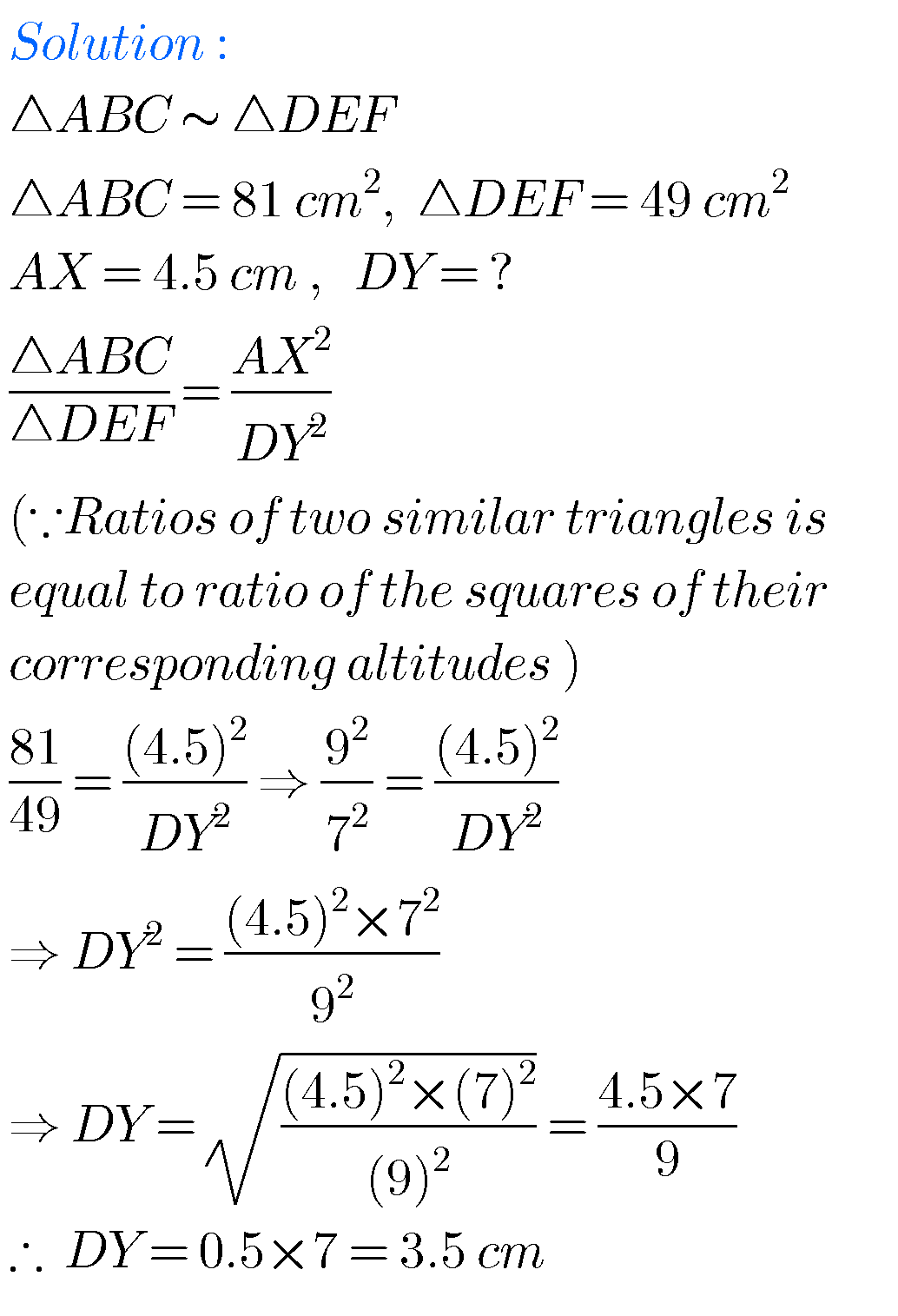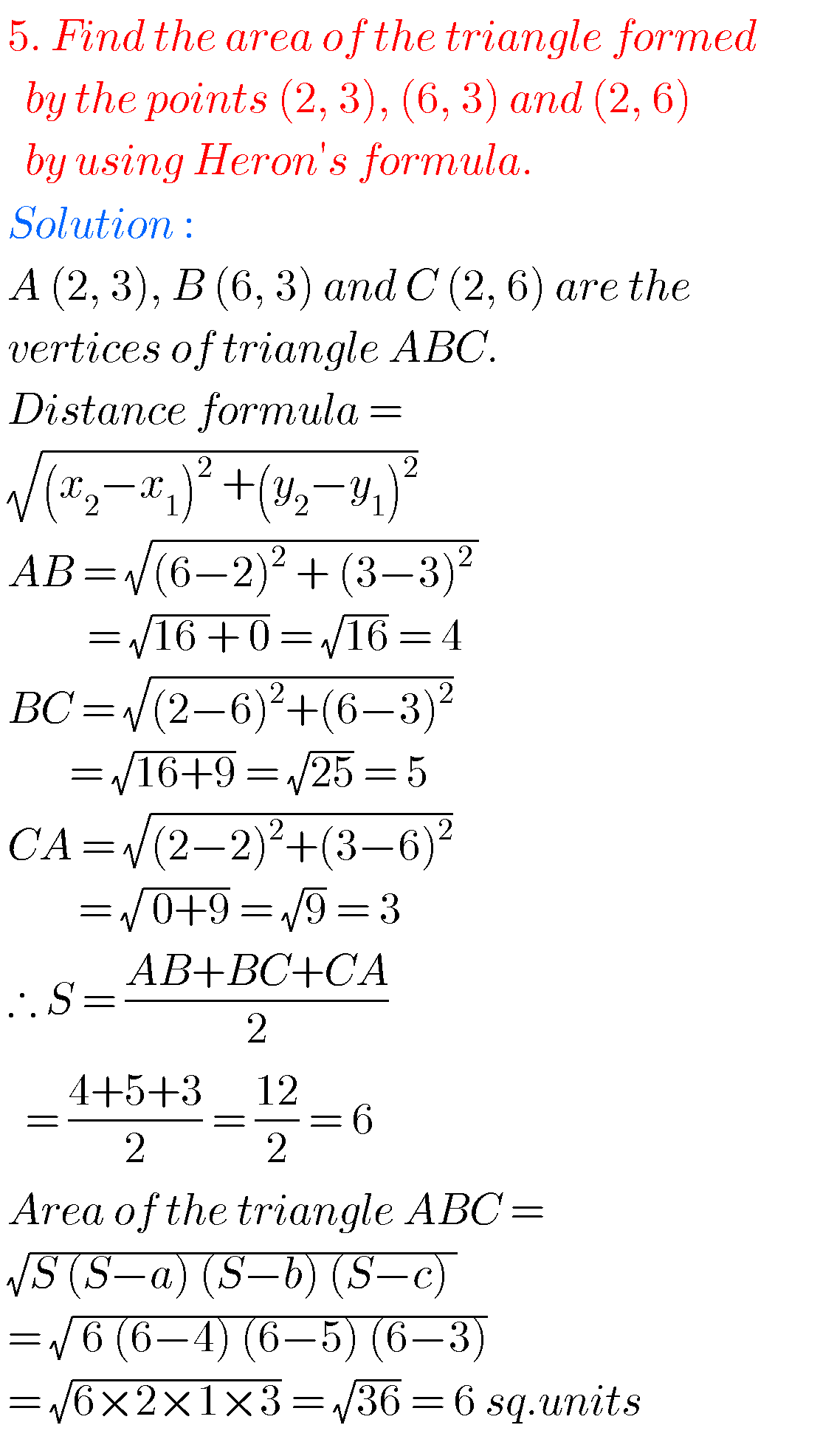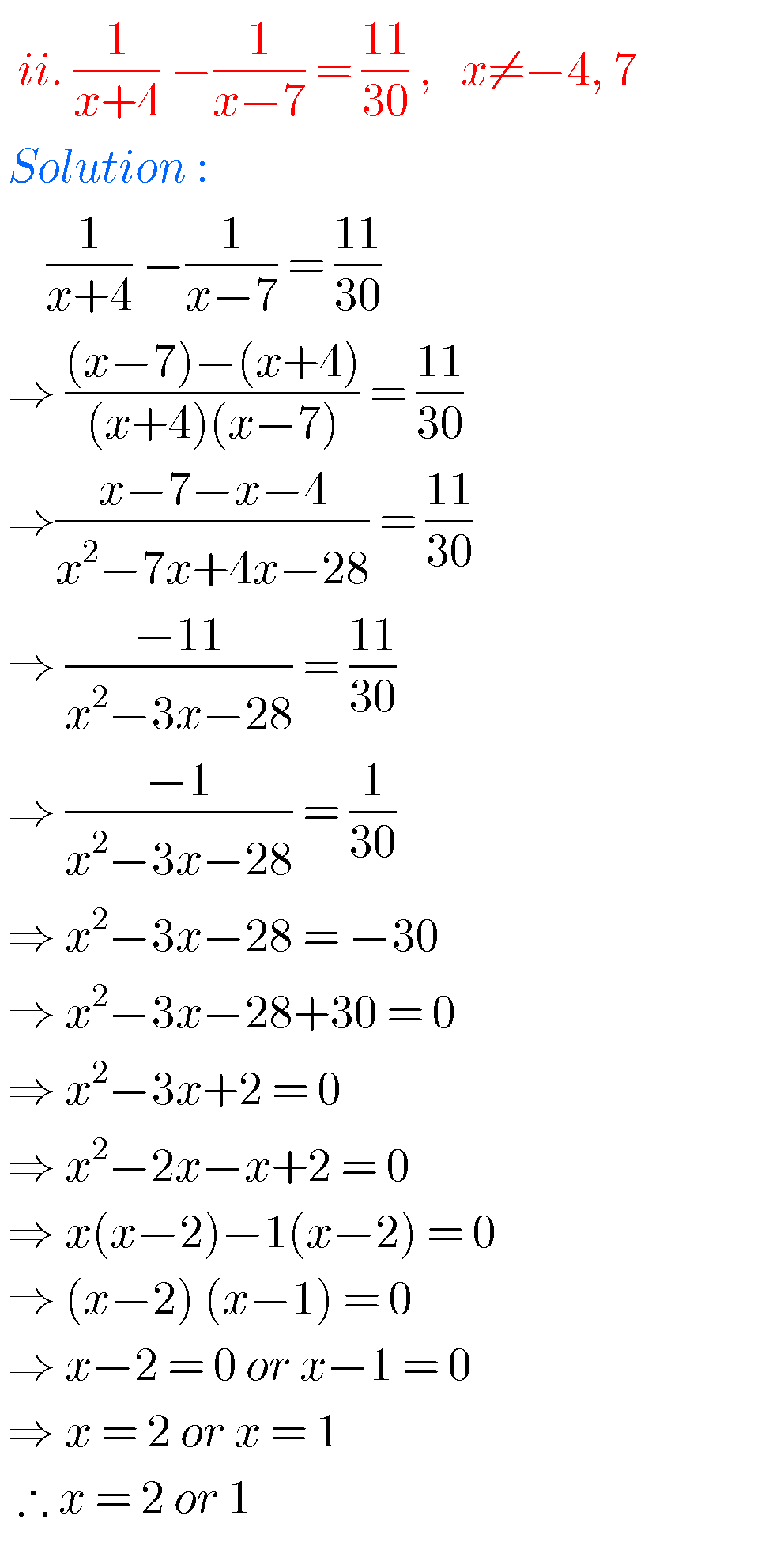# ssc maths class 10 solutions## Maths solutions for Applications of Trigonometry class 10 ssc

Maths solutions for Applications of Trigonometry class 10 Exercise 12.1 and12.2 Tenth class mathematics chapter 12 exercises 12.1 and 12.2 solutions are given. These solutions are very easy to understand. First study the chapter 12 well for many times. Observe the example probems and solutions well. Try them in your own method. Next solve the …## Maths solutions for Statistics class 10,SSC AP & TS Mathematics

Maths solutions for Statistics class 10 Exercise 8.1,8.2,8.3 and 8.4 Mathematics class 10 solutions for Statistics exercises 8,1, 8.2, 8.3 and 8.4 are given. These solutions are very easy to understand. First you study the lesson Statistics in the text book very well. Observe the example problems and solutions in the text book. You can …## Probability solutions class 10 maths SSC (AP & TS)

Probability solutions Class 10 Maths SSC Exercise 13.1 and 13.2 SSC mathematics class 10 Probability exercise 13.1 and exercise 13.2 solutions are given. Thsese are very easy to understand. First you study text book very well. Observe the example problems and solutions. Observe the solutions and try them in your own method. You can see …## SSC mathematics Trigonometry solutions class 10

Trigonometry solutions class 10 maths SSC Exercise 11.1,11.2,11.3 and 11.4 Tenth class mathematics chapter 11 Trigonometry exercises 11.1, 11.2, 11.3, 11.4, solutions are given. These solutions are very easy to understand. First you study textbook lesson Trigonometry very well. Practice example problems and solutions given in the textbook. Observe the given below solutions and try …## Tangents and Secants to a Circle solutions class 10 SSC,AP and TS Maths class X

Tangents and Secants to a Circle solutions class 10 Exercise 9.1,9.2,and 9.3 SSC Mathematics class 10 solutions for Tangents and Secants to a Circle exercises 9,1, 9.2 and 9.3 are given. These solutions are very easy to understand. First you study the lesson Tangents and Secands to a Circle in the text book very well. …## SSC solutions for Maths Similar Triangles class 10

SSC Class 10 solutions for maths Similar Triangles Exercise 8.1,8.2,8.3 and 8.4 Mathematics class 10 SSC Andhra predesh and Telangana Similar Triangles exercise 8.1, exercise 8.2, exercise 8.3 and exercise 8.4 solutions are given. These solutions are very easy to understand. First you study the text book lesson. Observe the example problems and solutions in …## SSC solutions for Maths Coordinate Geometry class 10, AP & TS

SSC Class 10 solutions for maths Coordinate Geometry Exercise 7.1,7.2,7.3 and 7.4 Mathematics solutions for Coordinate Geometry class 10 SSC. Exercise 7.1, exercise 7.2, exercise 7.3, and exercise 7.4 solutions are given. Thsese solutions are very easy to understand. First study the textbook lesson Coordinate Geometry very well. Observe the example problems and solutions given …## SSC solutions for Maths Progressions class 10

SSC Class 10 solutions for maths Progressions Exercise 6.1,6.2,6.3,6.4 and 6.5 Mathematics class 10 SSC Progressions text book problems with solutions are given. Exercises 6.1,6.2, 6.3,6.4 and 6.5. These are very easy to understand. First you study the text book very well. Observe the example problems and solutions given in the textbook. Observe the given …## SSC solutions for Maths Quadratic Equations class 10

SSC Class 10 solutions for Maths Quadratic Equations Exercise 5.1,5.2,5.3 and 5.4 Mathematics class 10 SSC solutions for Quadratic equations are given. Exercise 5.1, 5.2. 5.3 and 5.4 problems with solutions are given.  Observe the solutions and try them in your own method. First you study the text book lesson Quadtratic Equations very well.  Observe …## SSC Maths Solutions for Polynomials,Exercise 3.1, 3.2, 3.3, 3.4,Solutions for class 10 Maths chapter 3

Tenth maths guide for Polynomials, Exercise 3.1, 3.2, 3.3, 3.4. Tenth class mathematics solutions for text book problems. These are very easy to understand. Maths solutions for 10th class Andhra pradesh and Telangana. Study the textbook lesson Polynomials very well. Observe the given solutions and try them in your own method. You can also see …